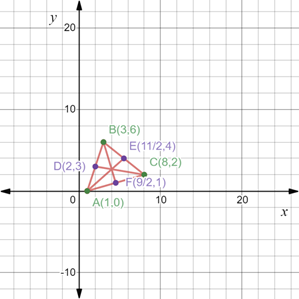# The lengths of the medians of the triangle with the vertices A ( 1 , 0 ) , B ( 3 , 6 ) and C ( 8 , 2 ) .### Precalculus: Mathematics for Calcu...

6th Edition
Stewart + 5 others
Publisher: Cengage Learning
ISBN: 9780840068071### Precalculus: Mathematics for Calcu...

6th Edition
Stewart + 5 others
Publisher: Cengage Learning
ISBN: 9780840068071

#### Solutions

Chapter 1.8, Problem 44E
To determine

## To calculate: The lengths of the medians of the triangle with the vertices A(1,0),B(3,6) and C(8,2) .

Expert Solution

The lengths of the medians of the triangle with vertices A(1,0),B(3,6) and C(8,2) are 1452,1092 and 37 .

### Explanation of Solution

Given information:

The three vertices of the triangle A(1,0),B(3,6) and C(8,2) .

Formula used:

Median of a triangle is defined as the line segment joining two points from a vertex to the midpoint of the opposite side.

Mid-point formula between two points X(a1,b1) and Y(a2,b2) is mathematically expressed as,

(a1+a22,b1+b22)

Distance formula between two points X(a1,b1) and Y(a2,b2) is calculated as,

d(XY)=(a2a1)2+(b2b1)2

Calculation:

Consider the provided vertices of the triangle A(1,0),B(3,6) and C(8,2) .

Recall the mid-point formula between two points X(a1,b1) and Y(a2,b2) is mathematically expressed as,

(a1+a22,b1+b22)

Let D, E and F be the mid-points of line segments AB, BC and CA respectively.

So, mid-point of line segment AB is calculated as,

D=(1+32,0+62)=(42,62)=(2,3)

Now, mid-point of line segment BC is calculated as,

E=(3+82,6+22)=(112,82)=(112,4)

Mid-point of line segment AC is calculated as,

F=(1+82,0+22)=(92,22)=(92,1)

By plotting all the points on the coordinate plane, we get the following figure,In the above figure, AE, BF and CD represents the three medians of the triangle ABC.

Recall the distance formula between two points X(a1,b1) and Y(a2,b2) is calculated as,

d(XY)=(a2a1)2+(b2b1)2

So, length of median AE is calculated as,

d(AE)=(1121)2+(40)2=(92)2+42=814+16=1452

Length of median BF is calculated as,

d(BF)=(923)2+(16)2=(32)2+(5)2=94+25=1092

Now, the length of median CD is calculated as,

d(CD)=(82)2+(23)2=(6)2+(1)2=36+1=37

Thus, the lengths of the medians of the triangle with vertices A(1,0),B(3,6) and C(8,2) are 1452,1092 and 37 .

### Have a homework question?

Subscribe to bartleby learn! Ask subject matter experts 30 homework questions each month. Plus, you’ll have access to millions of step-by-step textbook answers!